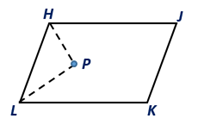Chapter 4.1, Problem 35E### Elementary Geometry for College St...

6th Edition
Daniel C. Alexander + 1 other
ISBN: 9781285195698

#### Solutions

Chapter
Section### Elementary Geometry for College St...

6th Edition
Daniel C. Alexander + 1 other
ISBN: 9781285195698
Textbook Problem
3 views

# The bisectors of two consecutive angles of ▱ HJKL are shown. What can you conclude regarding ∠P?To determine

To conclude:

The angle ∠P in the given parallelogram HJKL.

Explanation

Given:

The bisectors of two consecutive angles of HJKL are shown below.

Corollary:

A parallelogram is a quadrilateral in which both pairs of opposite sides are parallel.

In the given parallelogram IJKL.

 PROOF Statements Reasons 1. Parallelogram IJKL 1. Given 2. HJ-∥LK- 2. By the definition of a parallelogram 3. ∠LHP=∠H2 and ∠HLP=∠L2 3. Since HP and LP are angle bisectors of ∠H and ∠L 4

### Still sussing out bartleby?

Check out a sample textbook solution.

See a sample solution

#### The Solution to Your Study Problems

Bartleby provides explanations to thousands of textbook problems written by our experts, many with advanced degrees!

Get Started